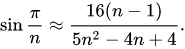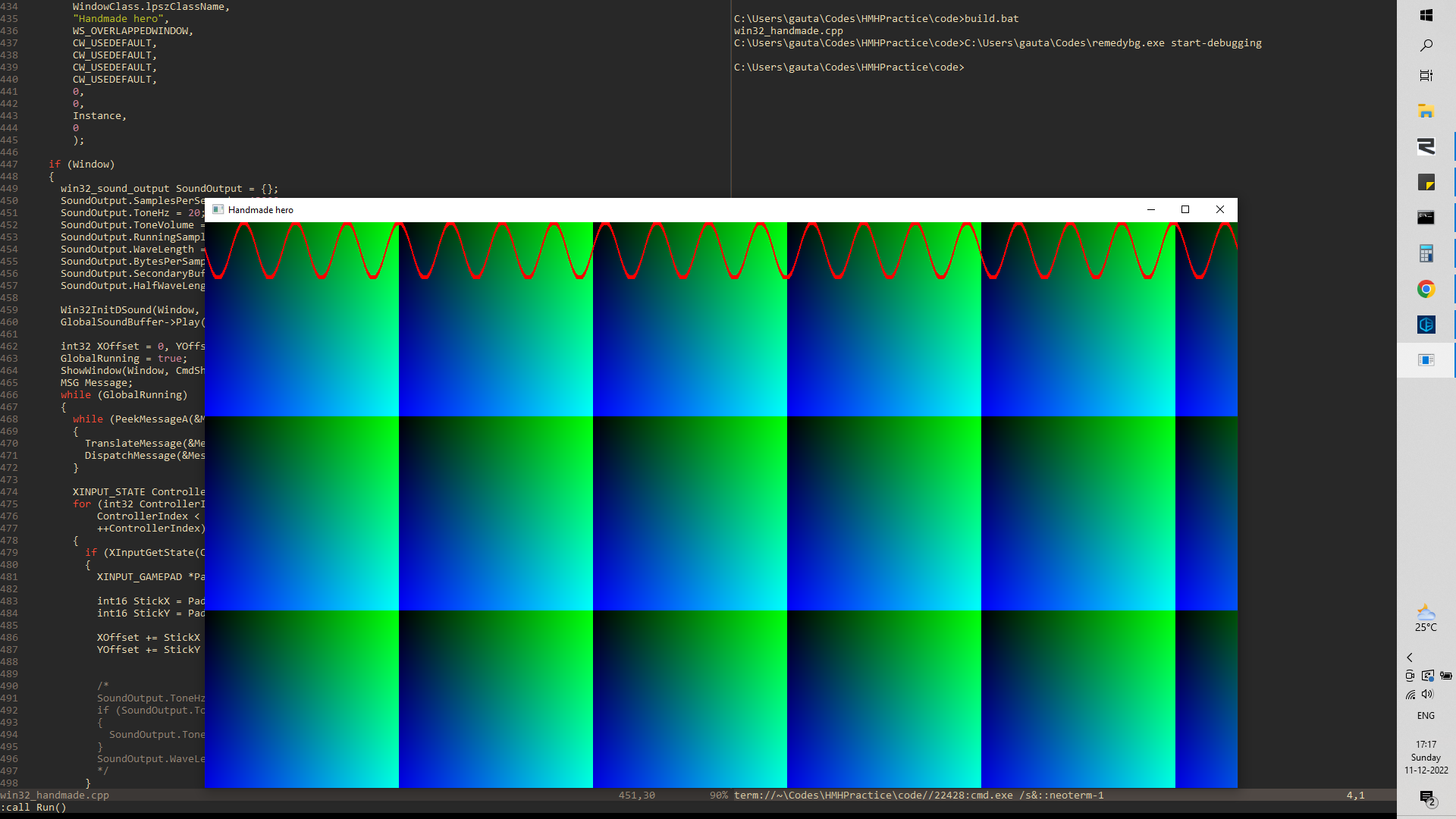Gaurav Gautam
96 posts
Was sine implemented from scratch in some episode?

I have been going over the first few days of HMH trying to get them in my head, and the TODO about implementing the sine catches my eye everytime I pass that section again. Did he implement the sine at some point in the future (maybe I have missed it if it happened in the first 100 or so episodes)?

Also, I went and googled how to do it from scratch and found some scary looking stuff. So apparently, its not as simple as just using some taylor series expansion and the way to do it is by an algorithm called the CORDIC explained here: https://www.allaboutcircuits.com/technical-articles/an-introduction-to-the-cordic-algorithm/

There also seems to be an x86 instruction which will compute the sine of a value referenced here: https://mudongliang.github.io/x86/html/file_module_x86_id_114.html

From the first article, I see that the basic idea is to rotate a unit vector aligned to the x-axis by the angle whose sine or cosine is needed and then read off the value of the rotated x or y coordinate. And this is fast because one can break down the angle of rotation into smaller angles such that those smaller angles always satisfy tan(x) = 2^(-i). Then, the problem reduces to division by powers of 2 which is just bit shifting. This leaves out a scaling factor which supposedly can be proved to be some fixed value within some error-bounds if we specify how many smaller angles we break our initial angle into.

Now, its unclear to me how that angle breaking part is done (even though the article provides a listing of one run with the values involved).

But I get the basic idea, i.e. implement this algorithm which takes out a fixed scaling factor (I'm definitely missing something here) that has the error inside it and the rest can be done using bit-shifts that are fast and don't have any error.

Does anyone know if

• the x86 instruction referenced in the second link just implements this algorithm?
• if the c runtime uses an implementation of this algorithm or just call the instruction in the hardware
• and whether his plan was to implement this algorithm on stream... man that math-hole would have been something to look at (or if it exists in some future episode tell me the episode number)
Gaurav Gautam
96 posts
Was sine implemented from scratch in some episode?

Please tell me he was just going to use the hardware instruction... this is a project in itself.

Mārtiņš Možeiko
2469 posts / 2 projects
Was sine implemented from scratch in some episode?
Edited by Mārtiņš Možeiko on

It was not implemented, and sinf from CRT is called in the code.

There is no "hardware instruction" for sine. I mean there was in x87 (the one you linked) but that is very obsolete and slow and no 64-bit code uses it anymore. It was relevant only on older 32-bit x86 CPU's.

To implemented sine in software you do not use CORDIC. Cordic is meant for hardware implementation. In software you do polynomial approximation which is much faster. You can tune precision based on what degree of polynomial you use.

If you want to take ready to use implementation, look here: http://gruntthepeon.free.fr/ssemath/ It has decent precision and reasonably fast implementation. It has been ported from cephes library: https://netlib.org/cephes/

To get good polynomial approximations in floating point you approximate maximum error over interval with taking into account floating point precision (single or double). You do not want to use Taylor expansion as that minimizes error around one point (where it is expanded) which means it can give very large error.

There are good algorithms that provide this approximation. It is called Remez algoritm. You can read about it in this pdf, which gives step-by-step explanation how to approximate sine: Even Faster Math Functions Start with page 64, but pages 24-57 about range reduction are good too.

It uses sollya math software and fpminimax command which does exactly what you want - gives polynomial approximation that minimizes maximum error in specified interval, and all the values take into account single/double precision floats.

You can also read few older presentations on function approximation from older entry in the same blog: Faster Math Functions

And if you don't care much about high precision approximation, and want to get just something very simple for your generic game code, then you can do some other tricks.
Like Bhaskara I's sine approximation formula
Or using just parabola and its square: https://web.archive.org/web/20060503192740/http://www.devmaster.net/forums/showthread.php?t=5784 Images are lost, but somebody on discord posted desmos graphs here: https://www.desmos.com/calculator/1okqgxknhc (look at blue vs violet lines).

If you want very accurate approximation, then this is a good resource: https://people.cs.rutgers.edu/~sn349/rlibm/

Simon Anciaux
1301 posts
Was sine implemented from scratch in some episode?
Edited by Simon Anciaux on

I believe this episodes (day 440) of handmade hero gives some explanations about how it work (not sure I haven't watch it in a while) https://www.youtube.com/watch?v=0b68cEY2wKs

Gaurav Gautam
96 posts
Was sine implemented from scratch in some episode?

Thankyou both for the links. I'm going through them :)

178 posts
Was sine implemented from scratch in some episode?

Regarding the Bhaskarasine formula, Is there any way for it to take turns (0-1 range) rather than radians or angles? The closest form of this in the wiki that I saw is:But it doesn't go from the 0 to 1 range.

Mārtiņš Možeiko
2469 posts / 2 projects
Was sine implemented from scratch in some episode?

The one in wikipedia does 0..360 range. So simply replace each x with x*360 and you'll get 0..1 range. The formula probably will simply a bit, because 40500 is a multiple of 180, so you could remove common factors from numerator and denumerator.

178 posts
Was sine implemented from scratch in some episode?

Regarding the parabola equation, I know that 1.27323954473516 is 4 / PI (based on Casey's Turns are Better than Radians blog). What does the number 0.40528473 represent? And if I use turns rather than radians, how would it change?

Mārtiņš Možeiko
2469 posts / 2 projects
Was sine implemented from scratch in some episode?
Edited by Mārtiņš Možeiko on

It's just derived from parabola equation. Look at that web archive link of devmaster. It explains how that was derived - 4/(pi*pi).

If you want to use turns there, just plug into quadratic equation known point coordinates on parabola in same way, and calculate quadratic equation coefficients from it.

Btw, on previous Bhaskarasine formula - there is no significant advantage of using 0..1 interval there. Because the equation does not change much. It matters more for polynomial approximations because then they can avoid of dividing by pi constant (as they can approximate directly with 0..1 argument). Otherwise be it radians or degrees, does not matter.

178 posts
Was sine implemented from scratch in some episode?

The link didn't work for me, but it does now. The author calculated sin in the range [-pi, pi] using abs. Is there any way to flip the curve for the range [pi, 2*pi] without remapping the input to [-pi, 0]?

Mārtiņš Možeiko
2469 posts / 2 projects
Was sine implemented from scratch in some episode?
Edited by Mārtiņš Možeiko on

abs is the operation that does remapping same parabola to 0..pi/2 just with negative sign in cases x>pi/2. You just need to figure out math that does remapping you want and it'll work to.

From top of my head, checking if x>pi/2 then doing subtract with pi/2 and flipping sign of result should do it. Write in C first, then optimize to sse, It should be just a few operations in sse. cmp,and,sub for input, then and,or for output, I think (have not really checked it). Or maybe just always subtracting pi/2 and flipping sign on result is better, not sure.

507 posts
Was sine implemented from scratch in some episode?

a cpysign at the end to get the result of the sin remapped beck from 0 - pi/2 to -pi/2 - 0 is a fairly simple operation

Gaurav Gautam
96 posts
Was sine implemented from scratch in some episode?
Edited by Gaurav Gautam on
Hey so while I can't say I understand all the material referenced here. I was visualizing the soundbuffer and I just threw in the bhaskara approximation for generating the sine wave. It works quite well:And I just used the theta instead of radians. And when the angle goes over 360 I just normalize it. And I use the identity sin(theta + pi) = -sin (theta)
I want to understand the other mathy stuff thats in the later episode as well as the links provided by martin. But this worked quite well for the uses of the sinf function so far. I think it will work well even for the coordinate rotation thats done a bit later based on the shape of the curve... I'll post the results when I get there.